ELECTROSTATICS II Electric Field 1 Electric Field 2

• Slides: 17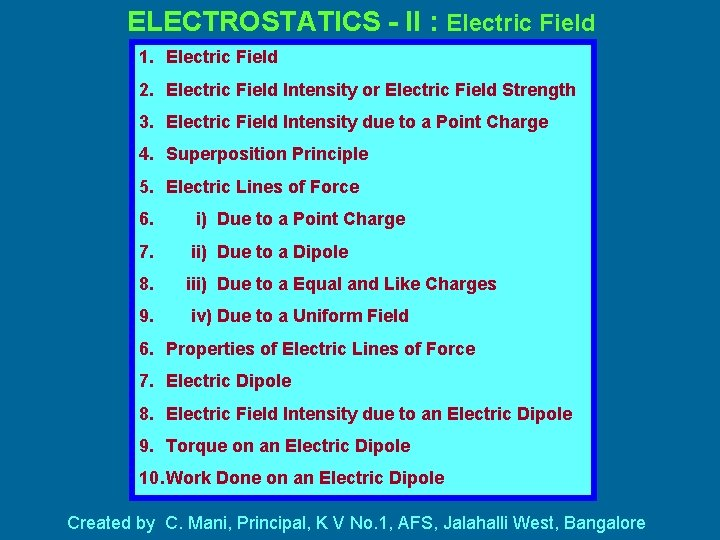ELECTROSTATICS - II : Electric Field 1. Electric Field 2. Electric Field Intensity or Electric Field Strength 3. Electric Field Intensity due to a Point Charge 4. Superposition Principle 5. Electric Lines of Force 6. i) Due to a Point Charge 7. ii) Due to a Dipole 8. iii) Due to a Equal and Like Charges 9. iv) Due to a Uniform Field 6. Properties of Electric Lines of Force 7. Electric Dipole 8. Electric Field Intensity due to an Electric Dipole 9. Torque on an Electric Dipole 10. Work Done on an Electric Dipole Created by C. Mani, Principal, K V No. 1, AFS, Jalahalli West, Bangalore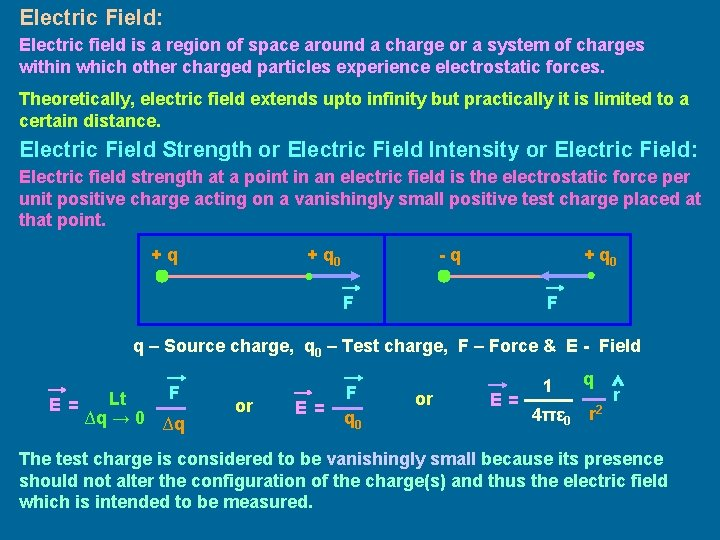Electric Field: Electric field is a region of space around a charge or a system of charges within which other charged particles experience electrostatic forces. Theoretically, electric field extends upto infinity but practically it is limited to a certain distance. Electric Field Strength or Electric Field Intensity or Electric Field: Electric field strength at a point in an electric field is the electrostatic force per unit positive charge acting on a vanishingly small positive test charge placed at that point. +q + q 0 -q + q 0 F F q – Source charge, q 0 – Test charge, F – Force & E - Field E= F Lt ∆q → 0 ∆q or E= F q 0 or E= 1 4πε 0 q r 2 r The test charge is considered to be vanishingly small because its presence should not alter the configuration of the charge(s) and thus the electric field which is intended to be measured.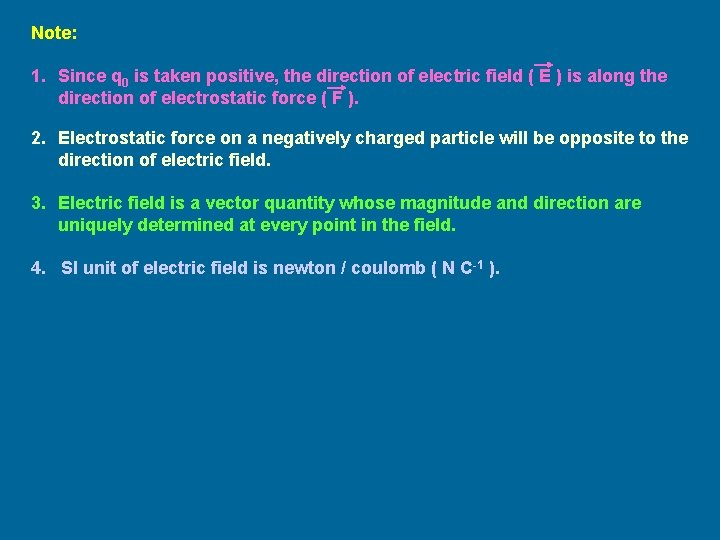Note: 1. Since q 0 is taken positive, the direction of electric field ( E ) is along the direction of electrostatic force ( F ). 2. Electrostatic force on a negatively charged particle will be opposite to the direction of electric field. 3. Electric field is a vector quantity whose magnitude and direction are uniquely determined at every point in the field. 4. SI unit of electric field is newton / coulomb ( N C-1 ).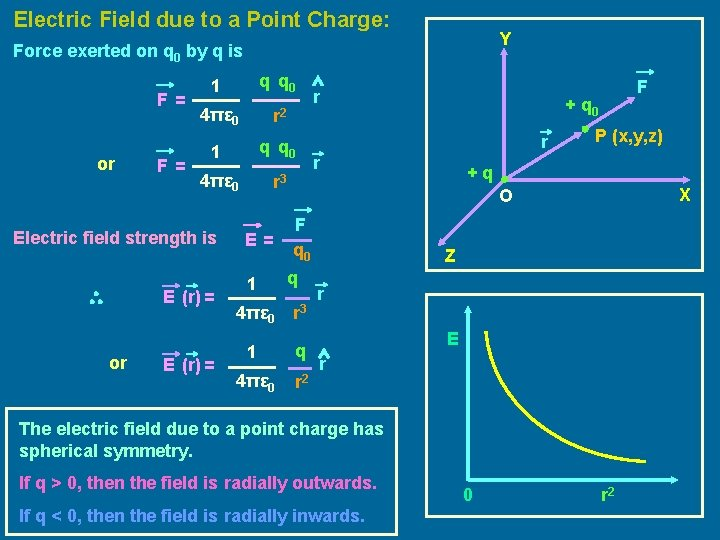Electric Field due to a Point Charge: Y Force exerted on q 0 by q is F= or F= 1 q q 0 4πε 0 r 2 1 q q 0 4πε 0 r 3 Electric field strength is E (r) = or E (r) = E= 1 r F + q 0 r r P (x, y, z) +q X O F q 0 q 4πε 0 r 3 1 q 4πε 0 r 2 Z r E r The electric field due to a point charge has spherical symmetry. If q > 0, then the field is radially outwards. If q < 0, then the field is radially inwards. 0 r 2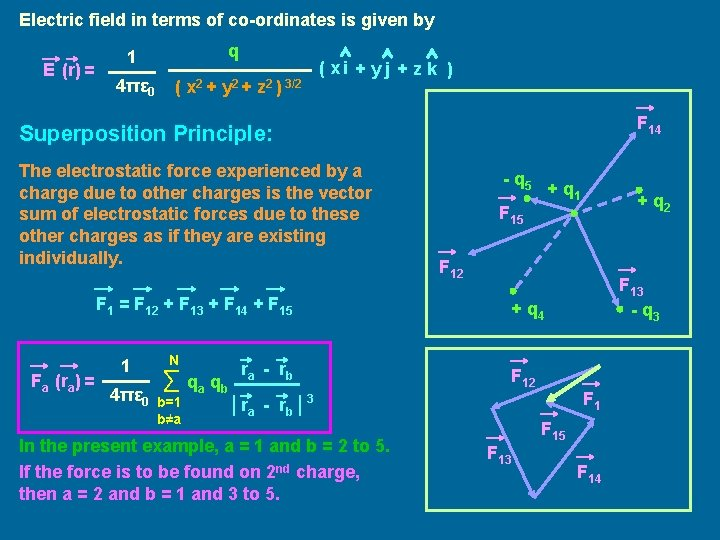Electric field in terms of co-ordinates is given by E (r) = q 1 4πε 0 ( x 2 + y 2 + z 2 ) 3/2 ( xi + y j + z k ) F 14 Superposition Principle: The electrostatic force experienced by a charge due to other charges is the vector sum of electrostatic forces due to these other charges as if they are existing individually. F 1 = F 12 + F 13 + F 14 + F 15 Fa (ra) = 1 4πε 0 N ∑ qa qb b=1 b≠a ra - rb - q 5 + q 1 F 12 F 13 - q 3 + q 4 F 12 F 1 │ ra - rb │ 3 In the present example, a = 1 and b = 2 to 5. If the force is to be found on 2 nd charge, then a = 2 and b = 1 and 3 to 5. + q 2 F 15 F 13 F 14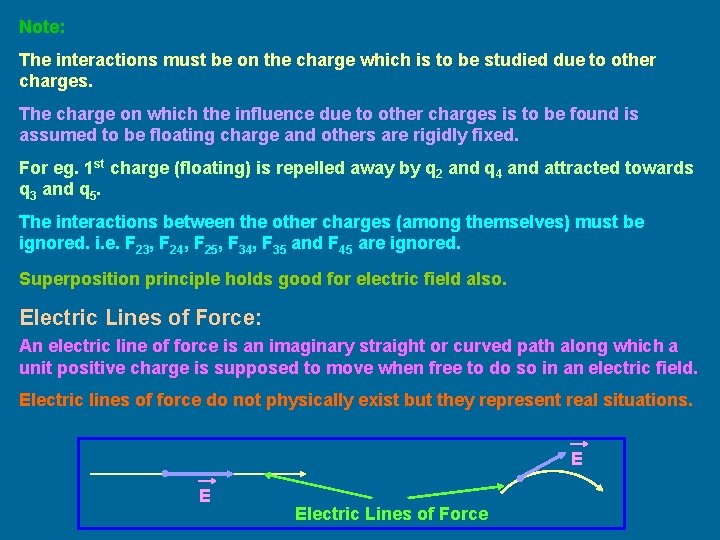Note: The interactions must be on the charge which is to be studied due to other charges. The charge on which the influence due to other charges is to be found is assumed to be floating charge and others are rigidly fixed. For eg. 1 st charge (floating) is repelled away by q 2 and q 4 and attracted towards q 3 and q 5. The interactions between the other charges (among themselves) must be ignored. i. e. F 23, F 24, F 25, F 34, F 35 and F 45 are ignored. Superposition principle holds good for electric field also. Electric Lines of Force: An electric line of force is an imaginary straight or curved path along which a unit positive charge is supposed to move when free to do so in an electric field. Electric lines of force do not physically exist but they represent real situations. E E Electric Lines of Force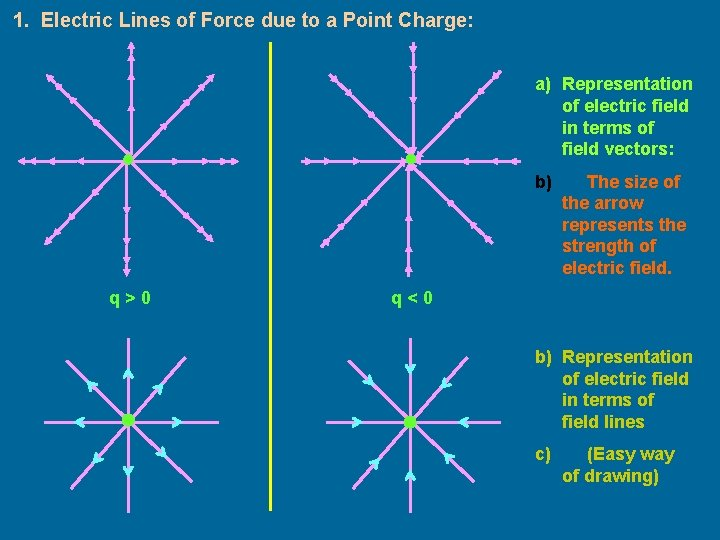1. Electric Lines of Force due to a Point Charge: a) Representation of electric field in terms of field vectors: b) q>0 The size of the arrow represents the strength of electric field. q<0 b) Representation of electric field in terms of field lines c) (Easy way of drawing)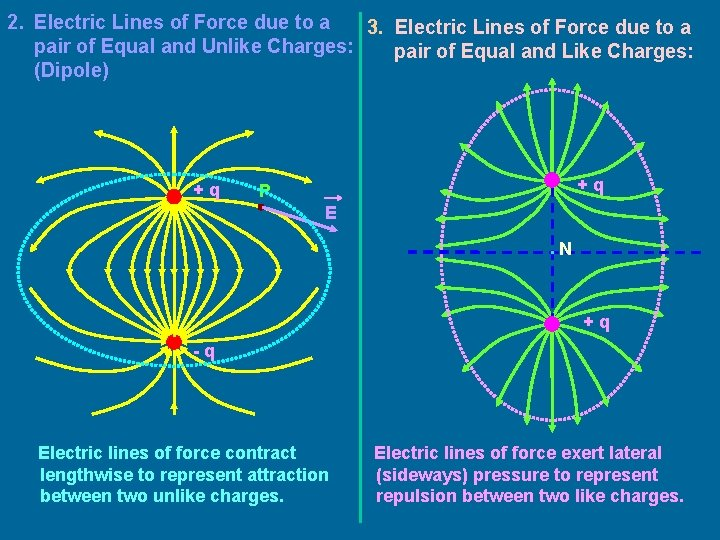2. Electric Lines of Force due to a 3. Electric Lines of Force due to a pair of Equal and Unlike Charges: pair of Equal and Like Charges: (Dipole) +q +q P E . N +q -q Electric lines of force contract lengthwise to represent attraction between two unlike charges. Electric lines of force exert lateral (sideways) pressure to represent repulsion between two like charges.4. Electric Lines of Force due to a Uniform Field: Properties of Electric Lines of Force or Field Lines: E - + + +1 C 1. The electric lines of force are imaginary lines. 2. A unit positive charge placed in the electric field tends to follow a path along the field line if it is free to do so. 3. The electric lines of force emanate from a positive charge and terminate on a negative charge. . 4. The tangent to an electric field line at any point gives the direction of the electric field at that point. 5. Two electric lines of force can never cross each other. If they do, then at the point of intersection, there will be two tangents. It means there are two values of the electric field at that point, which is not possible. Further, electric field being a vector quantity, there can be only one resultant field at the given point, represented by one tangent at the given point for the given line of force. E P E 1 S S O E L IB E N P T O E 2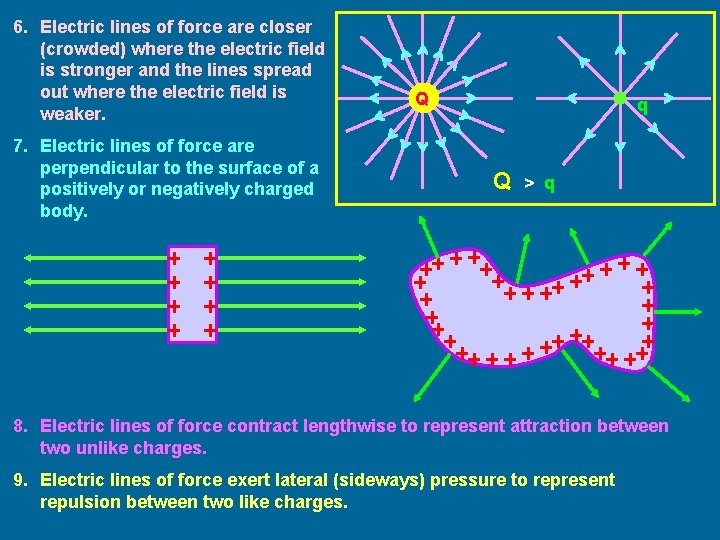6. Electric lines of force are closer (crowded) where the electric field is stronger and the lines spread out where the electric field is weaker. 7. Electric lines of force are perpendicular to the surface of a positively or negatively charged body. Q q Q > q 8. Electric lines of force contract lengthwise to represent attraction between two unlike charges. 9. Electric lines of force exert lateral (sideways) pressure to represent repulsion between two like charges.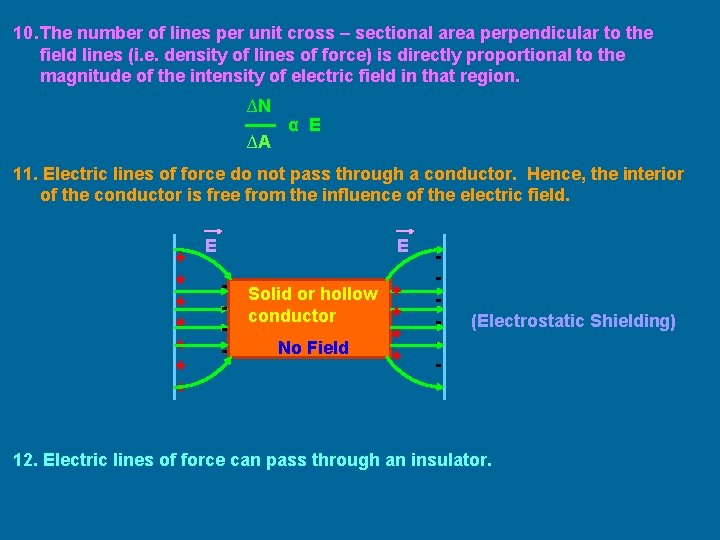10. The number of lines per unit cross – sectional area perpendicular to the field lines (i. e. density of lines of force) is directly proportional to the magnitude of the intensity of electric field in that region. ∆N ∆A α E 11. Electric lines of force do not pass through a conductor. Hence, the interior of the conductor is free from the influence of the electric field. + + + + E E - Solid or hollow + + conductor + No Field + - (Electrostatic Shielding) 12. Electric lines of force can pass through an insulator.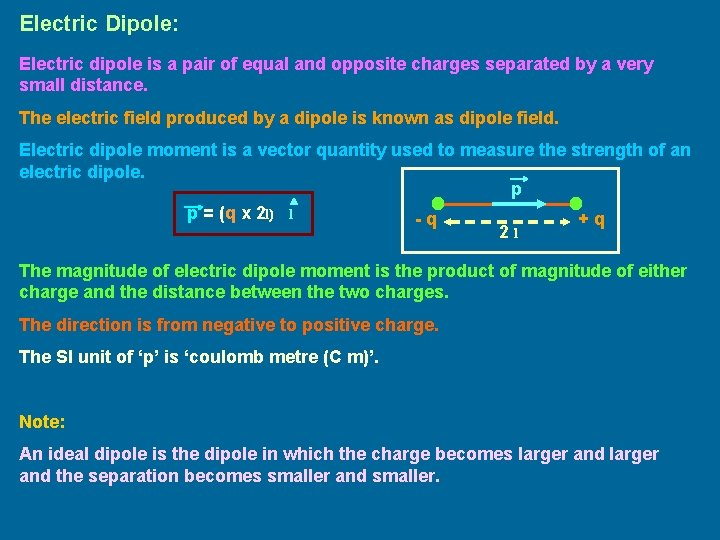Electric Dipole: Electric dipole is a pair of equal and opposite charges separated by a very small distance. The electric field produced by a dipole is known as dipole field. Electric dipole moment is a vector quantity used to measure the strength of an electric dipole. p p = (q x 2 l) l -q +q 2 l The magnitude of electric dipole moment is the product of magnitude of either charge and the distance between the two charges. The direction is from negative to positive charge. The SI unit of ‘p’ is ‘coulomb metre (C m)’. Note: An ideal dipole is the dipole in which the charge becomes larger and the separation becomes smaller and smaller.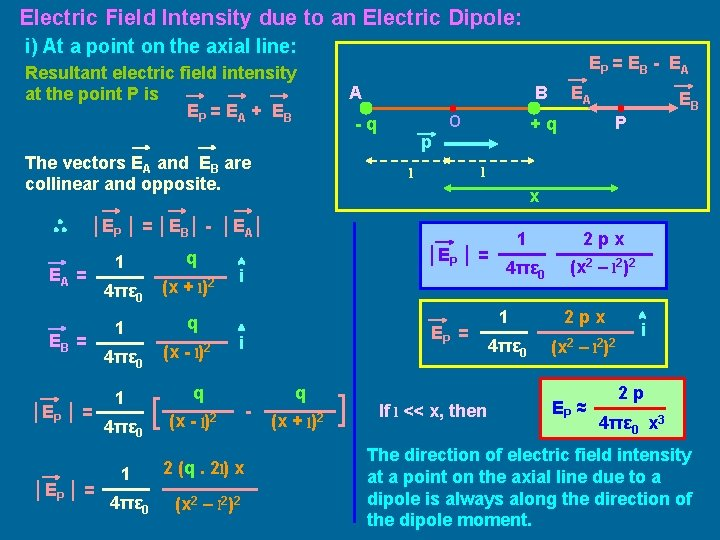Electric Field Intensity due to an Electric Dipole: i) At a point on the axial line: EP = EB - E A Resultant electric field intensity at the point P is EP = EA + E B A B O -q The vectors EA and EB are collinear and opposite. l EA = EB = │EP │ = q 4πε 0 (x + l)2 1 q 4πε 0 1 4πε 0 (x - l)2 EP = i q [ (x - l) │EP │ = i 2 1 2 (q. 2 l) x 4πε 0 (x 2 – l 2)2 - q (x + l)2 ] P x │EP │ = │EB│ - │EA│ 1 EB +q p l EA If l << x, then 1 2 px 4πε 0 (x 2 – l 2)2 1 4πε 0 2 px (x 2 – EP ≈ l 2 )2 i 2 p 4πε 0 x 3 The direction of electric field intensity at a point on the axial line due to a dipole is always along the direction of the dipole moment.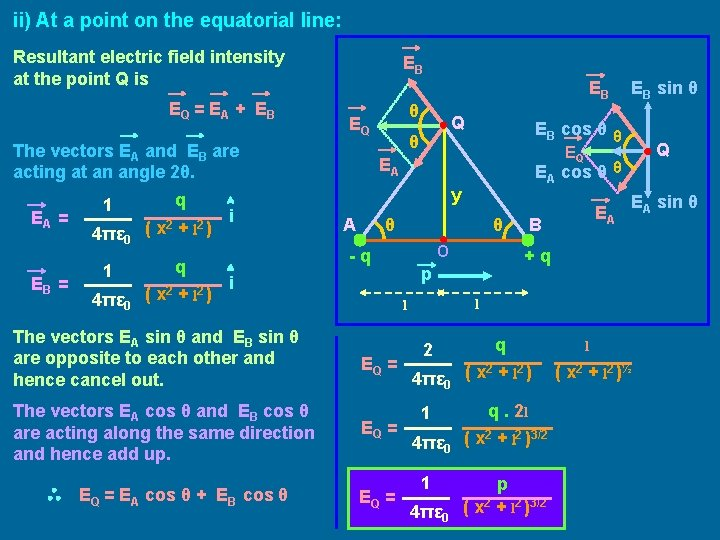ii) At a point on the equatorial line: Resultant electric field intensity at the point Q is EQ = EA + E B EB EA = EB = 2 2 4πε 0 ( x + l ) q 1 4πε 0 ( x 2 + Q θ l 2 ) i EA cos θ θ O -q The vectors EA sin θ and EB sin θ are opposite to each other and hence cancel out. The vectors EA cos θ and EB cos θ are acting along the same direction and hence add up. EQ = EA cos θ + EB cos θ EQ = B EA sin θ l l EQ = EA +q p i Q EQ EA A EB sin θ EB cos θ θ y q 1 θ EQ The vectors EA and EB are acting at an angle 2θ. EB 2 q 2 2 4πε 0 ( x + l ) 1 q. 2 l 2 2 3/2 4πε 0 ( x + l ) 1 4πε 0 p ( x 2 + l 2 )3/2 l ( x 2 + l 2 )½EQ = 1 4πε 0 p ( x 2 + l 2 )3/2 (- i ) If l << y, then EQ ≈ p 4πε 0 y 3 The direction of electric field intensity at a point on the equatorial line due to a dipole is parallel and opposite to the direction of the dipole moment. If the observation point is far away or when the dipole is very short, then the electric field intensity at a point on the axial line is double the electric field intensity at a point on the equatorial line. i. e. If l << x and l << y, then EP = 2 EQTorque on an Electric Dipole in a Uniform Electric Field: The forces of magnitude p. E act opposite to each other and hence net force acting on the dipole due to external uniform electric field is zero. So, there is no translational motion of the dipole. 2 l q. E θ q. E p -q E However the forces are along different lines of action and constitute a couple. Hence the dipole will rotate and experience torque. Torque = Electric Force x t = q E (2 l sin θ) +q p θ distance E t = p E sin θ t = px. E Direction of Torque is perpendicular and into the plane containing p and E. SI unit of torque is newton metre (Nm). Case i: If θ = 0°, then t = 0. Case ii: If θ = 90°, then t = p. E (maximum value). Case iii: If θ = 180°, then t = 0.Work done on an Electric Dipole in Uniform Electric Field: When an electric dipole is placed in a uniform electric field, it experiences torque and tends to allign in such a way to attain stable equilibrium. d. W = tdθ q. E dθ + q q E = p E sin θ dθ θ 2 W = ∫ p E sin θ dθ θ 1 2 l q. E -q θ 1 θ 2 E W = p E (cosθ 1 - cos θ 2) If Potential Energy is arbitrarily taken zero when the dipole is at 90°, then P. E in rotating the dipole and inclining it at an angle θ is Potential Energy U = - p E cos θ Note: Potential Energy can be taken zero arbitrarily at any position of the dipole. Case i: If θ = 0°, then U = - p. E (Stable Equilibrium) Case ii: If θ = 90°, then U = 0 Case iii: If θ = 180°, then U = p. E (Unstable Equilibrium) END